How to place OP-AMP (741) on the bread board?FIGURE # 1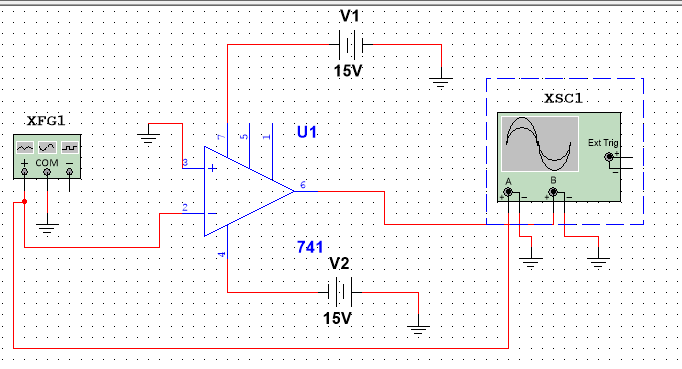FIGURE #1 (waveform result)FIGURE # 2
If we set R2 = 10 k ohm...If we set R2 = 20 k ohm...
As you can see, when R2 increase, Vo increase.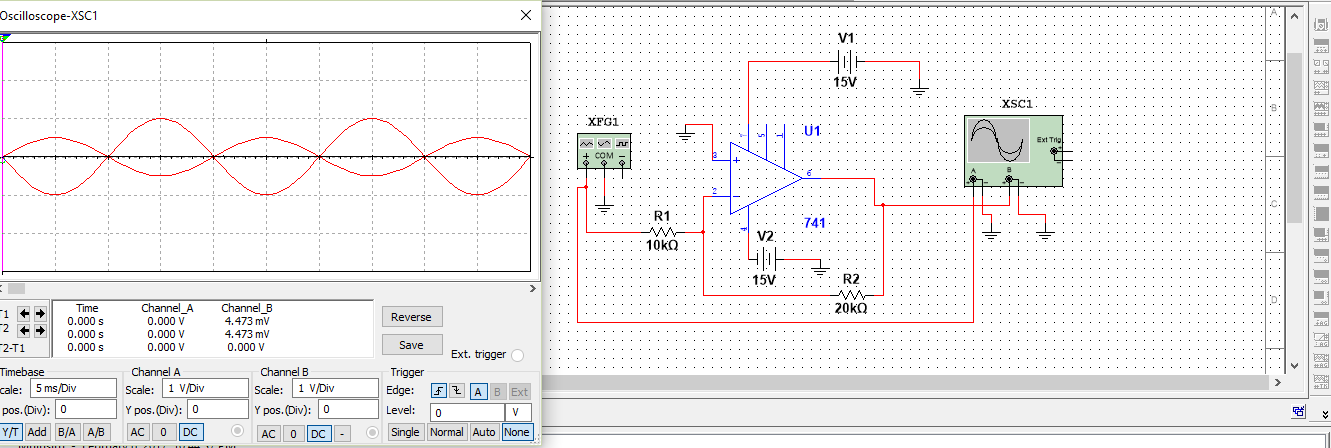Based on that, to get Vo/Vi = -10,
the correct answer for R2 is 100k ohm

FIGURE # 3
gate #3 connect to FG, gate #2 connect to resisotr and ground. Note: The correct value for R2 is 100 k ohm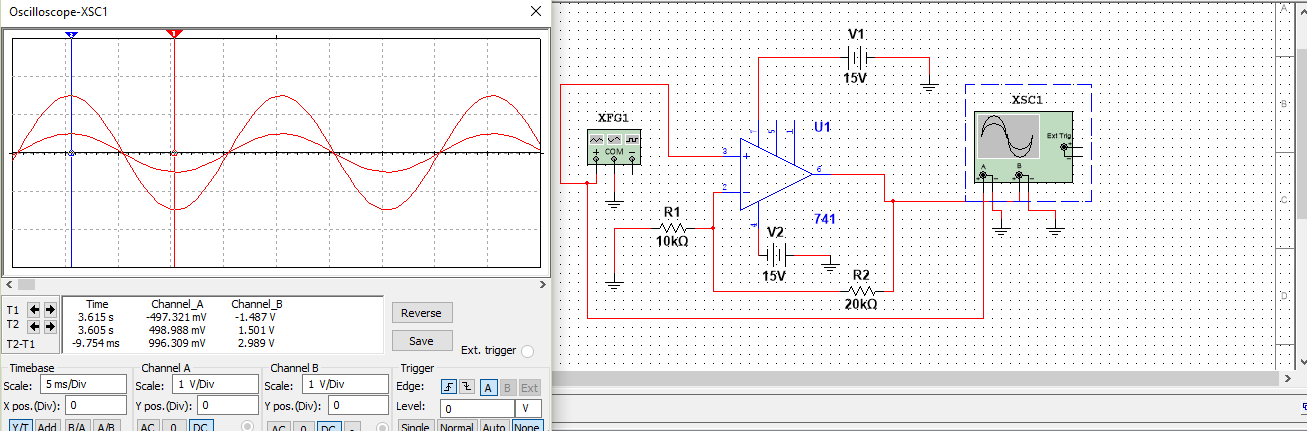FIGURE # 4a4a ResultFIGURE # 4b4b Result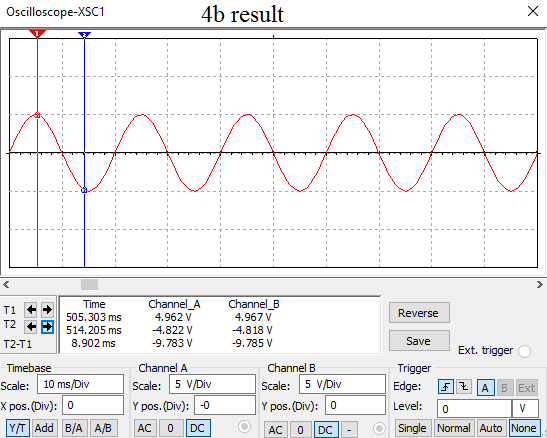FIGURE # 5
Voltage Divider (use 3 x 10k ohm resistors)CASE : When Rx = 100 ohm.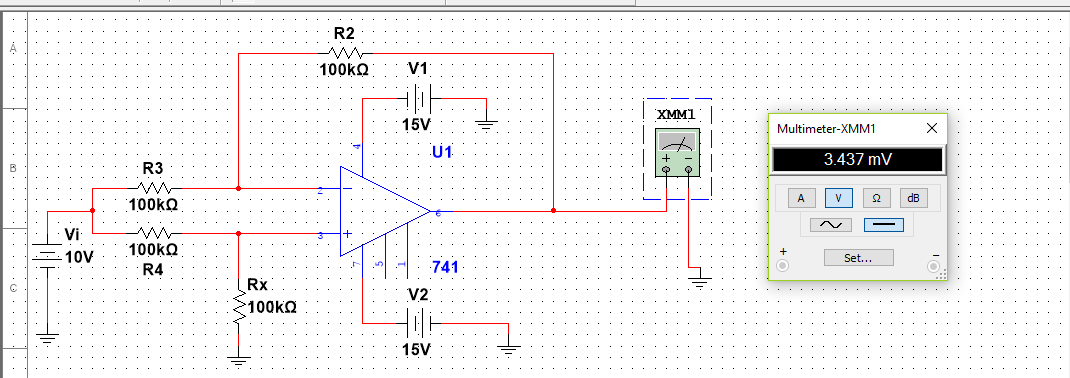CASE : When Rx = 0 ohm (short circuit).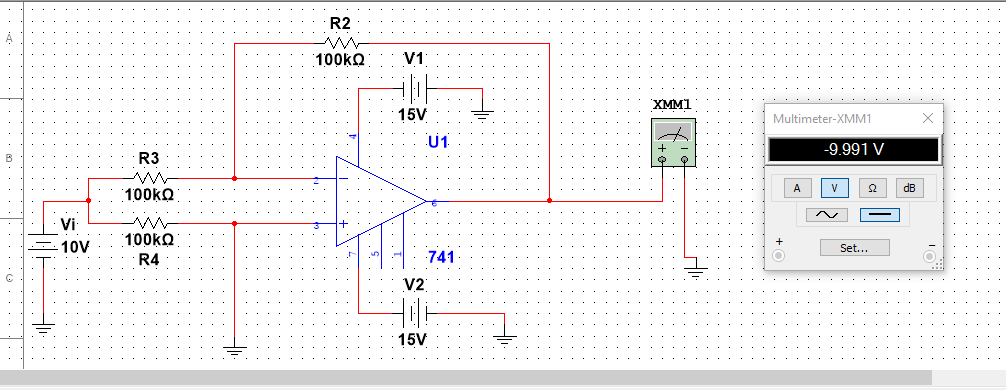CASE : When Rx = ∞ ohm (open circuit).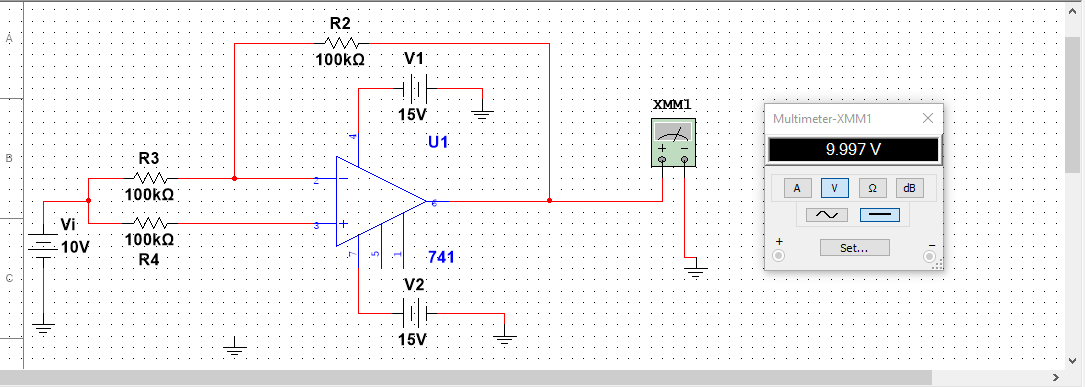I will prepared a midterm review if possible, wish you all the best for the midterm.

Kevin Tang (ktang@ryerson.ca)